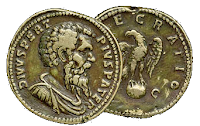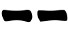## Sunday, 23 January 2022Another method, with only two coins, I learned from Clarity

1. Throw the two coins.
If they both show head count 2 otherwise count 3
2. Throw the two cons again
Count 2 for each head and 3 for each tail.
3. Sum it all up and draw the resulting line according the following table:
 6 7 8 94. Repeat steps 1 and 2 other five times drawing the resulting lines from the bottom to the top of the hexagram.

#### Probabilities

This method generates lines with the same probabilities as the yarrow stalk method because the odds of getting 2 in the first throw are 1/4.

For each of the possible eight outcomes, probabilities are:

 2+2+2 = 61/4 * 1/2 * 1/2 = 1/16 2+2+3 = 71/4 * 1/2 * 1/2 = 1/16 2+3+2 = 71/4 * 1/2 * 1/2 = 1/16 2+3+3 = 81/4 * 1/2 * 1/2 = 1/16 3+2+2 = 73/4 * 1/2 * 1/2 = 3/16 3+2+3 = 83/4 * 1/2 * 1/2 = 3/16 3+3+2 = 83/4 * 1/2 * 1/2 = 3/16 3+3+3 = 93/4 * 1/2 * 1/2 = 3/16

Meaning that:

Prob(6) = 1/16
Prob(8) = 7/16 = 1/16 + 3/16 + 3/16
Prob(7) = 5/16 = 1/16 + 1/16 + 3/16
Prob(9) = 3/16
Prob(yin) = Prob(yang) = 1/2